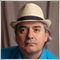Understanding RSI calculation126

Hey, need your expertize!

Question in short: SHOULD I NOT INCLUDE THE CURRENT BARS CHANGEUP/CHANGEDOWN WHEN CALCULATING THE RSI FOR THAT PARTICULAR BAR?

Question in long form:

I need some help understanding the what to include/exclude in calculating RSI for different amounts or RSI bars, say 10 and 14 as an example.

This is for a C# programming issue so I would like to just evaluate my thoughts, not rearrange them unless absolut necessary.

1) First I look at gain/loss per bar for a timeperiod that contains maybe 1000 bars.

I go through each bar, setting bar(gain&loss or ChangeUp/ChangeDown) to = close price for CURRENT BAR compared to close for PREVIOUS BAR.  If change is positive I set ChangeUp to CURRENTBARclosePrice - PREVIOUSBARclosePrice and ChangeDown is set to 0.
Vice versa if the change would be negative.
I make sure that ChangeUp/ChangeDown is always a positive number.

2) Then to find RSI for a particular amount of RSIbars I add all the ChangeUp and then add all ChangeUp for the amount of RSI bars and divide them by amounts of RSIbars (like 10 in my example)

AvgU = totalChangeUp / NoOfBars;

AvgD = totalChangeDown / NoOfBars;

then do RS = AvgU/AvgD

then do RSI = (100 - (100 / (1 + RS)))

So if I have 10 in my RSI setting for bars. I add the 10 latest bar INCLUDING THE CURRENT BAR to get the RSI for that particular bar.

SHOULD I NOT INCLUDE THE CURRENT BARS CHANGEUP/CHANGEDOWN WHEN CALCULATING THE RSI FOR THAT PARTICULAR BAR?4946

d.Johan StrandbergQuestion in short: SHOULD I NOT INCLUDE THE CURRENT BARS CHANGEUP/CHANGEDOWN WHEN CALCULATING THE RSI FOR THAT PARTICULAR BAR?

Question in long form:

I need some help understanding the what to include/exclude in calculating RSI for different amounts or RSI bars, say 10 and 14 as an example.

This is for a C# programming issue so I would like to just evaluate my thoughts, not rearrange them unless absolut necessary.

1) First I look at gain/loss per bar for a timeperiod that contains maybe 1000 bars.

I go through each bar, setting bar(gain&loss or ChangeUp/ChangeDown) to = close price for CURRENT BAR compared to close for PREVIOUS BAR.  If change is positive I set ChangeUp to CURRENTBARclosePrice - PREVIOUSBARclosePrice and ChangeDown is set to 0.
Vice versa if the change would be negative.
I make sure that ChangeUp/ChangeDown is always a positive number.

2) Then to find RSI for a particular amount of RSIbars I add all the ChangeUp and then add all ChangeUp for the amount of RSI bars and divide them by amounts of RSIbars (like 10 in my example)

AvgU = totalChangeUp / NoOfBars;

AvgD = totalChangeDown / NoOfBars;

then do RS = AvgU/AvgD

then do RSI = (100 - (100 / (1 + RS)))

So if I have 10 in my RSI setting for bars. I add the 10 latest bar INCLUDING THE CURRENT BAR to get the RSI for that particular bar.

SHOULD I NOT INCLUDE THE CURRENT BARS CHANGEUP/CHANGEDOWN WHEN CALCULATING THE RSI FOR THAT PARTICULAR BAR?

Have a look at WikiPedia's page on RSI (Relative Strength Indicator) which offers you a complete explanation of how it is calculated.

You can also consult the original source, which is the 1978 book by J. Welles Wilder, New Concepts in Technical Trading Systems, that has an example step-by-step breakdown on how it is calculated.19857

Look at the actual RSI (MT4) code.
diff=close[i]-close[i-1];
ExtPosBuffer[i]=(ExtPosBuffer[i-1]*(InpRSIPeriod-1)+(diff>0.0?diff:0.0))/InpRSIPeriod;
ExtNegBuffer[i]=(ExtNegBuffer[i-1]*(InpRSIPeriod-1)+(diff<0.0?-diff:0.0))/InpRSIPeriod;
if(ExtNegBuffer[i]!=0.0)
ExtRSIBuffer[i]=100.0-100.0/(1+ExtPosBuffer[i]/ExtNegBuffer[i]);

It's not defined as totalChangeUp / NoOfBars. It's a EMA(2/InpRSIPeriod -1) of the current bar only.126

Fernando Carreiro:

Have a look at WikiPedia's page on RSI (Relative Strength Indicator) which offers you a complete explanation of how it is calculated.

You can also consult the original source, which is the 1978 book by J. Welles Wilder, New Concepts in Technical Trading Systems, that has an example step-by-step breakdown on how it is calculated.

You do know that RSI AveageUp and AverageDown can be calculated using SMA, EMA and Wilders Smooting right?

Just asking as your answer did not contain anything of value to my question that google had not already provided.....126

whroeder1:
Look at the actual RSI (MT4) code.

It's not defined as totalChangeUp / NoOfBars. It's a EMA(2/InpRSIPeriod -1) of the current bar only.

Awesome, whroeder1.
As stated in my doublethread already. If I want my code to mimic the indicator in MT4 I need to comply with the AverageUp and AverageDown-calculation of it, i.e. using EMA instead of SMA.

I guess not that many people ever use SMA in their calculations of RSI and EMA is standard. Think I'll change my code to EMA now :)